## RS Aggarwal Class 9 Solutions Chapter 9 Quadrilaterals and Parallelograms Ex 9C

These Solutions are part of RS Aggarwal Solutions Class 9. Here we have given RS Aggarwal Class 9 Solutions Chapter 9 Quadrilaterals and Parallelograms Ex 9C.

Other Exercises

Question 1.
Solution:
Given : In trapezium ABCD,
AB || DC and E is the midpoint of AD.
A line EF ||AB is drawn meeting BC at F.
To prove : F is midpoint of BC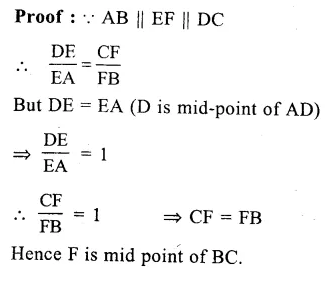Question 2.
Solution:
Given : In ||gm ABCD, E and F are the mid points of AB and CD respectively. A line segment GH is drawn which intersects AD, EF and BC at G, P and H respectively.
To prove : GP = PH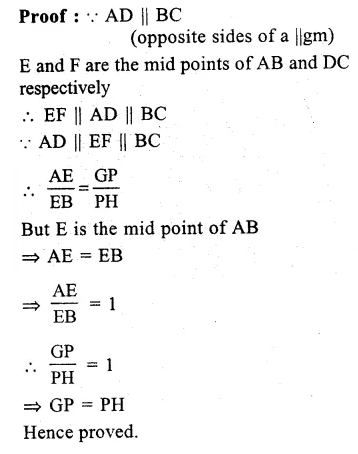Question 3.
Solution:
Given : In trapezium ABCD, AB || DC
P, Q are the midpoints of sides AD and BC respectively
DQ is joined and produced to meet AB produced at E
Join AC which intersects PQ at R.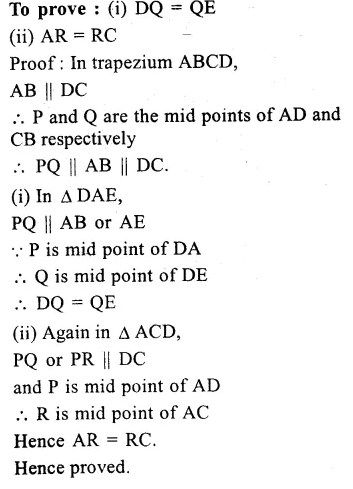Question 4.
Solution:
Given : In ∆ ABC,
AD is the mid point of BC
DE || AB is drawn. BE is joined.
To prove : BE is the median of ∆ ABC.
Proof : In ∆ ABC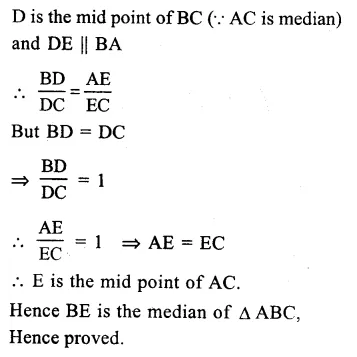Question 5.
Solution:
Given : In ∆ ABC, AD and BE are the medians. DF || BE is drawn meeting AC at F.
To prove : CF = $$\frac { 1 }{ 4 }$$ BC.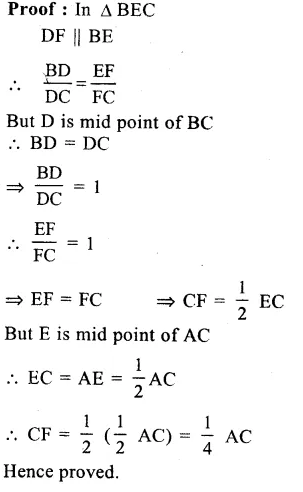Question 6.
Solution:
Given : In ||gm ABCD, E is mid point of DC.
EB is joined and through D, DEG || EB is drawn which meets CB produced at G and cuts AB at F.
To prove : (i)AD = $$\frac { 1 }{ 2 }$$ GC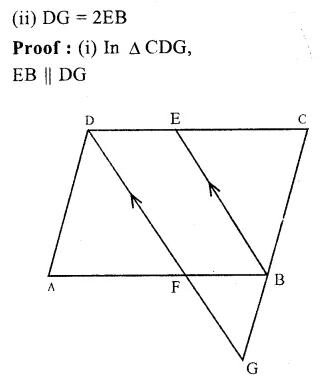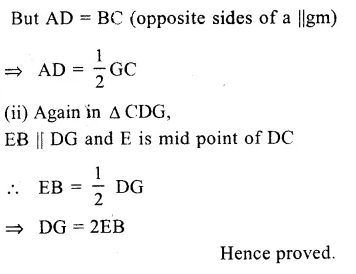Question 7.
Solution: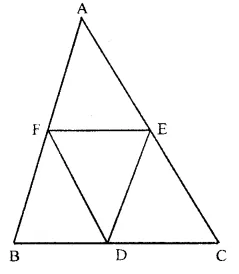Given : In ∆ ABC,
D, E and F are the mid points of sides BC, CA and AB respectively
DE, EF and FD are joined.Question 8.
Solution:
Given : In ∆ ABC, D, E and F are the mid points of sides BC, CA and AB respectivelyQuestion 9.
Solution:
Given : In rectangle ABCD, P, Q, R and S are the midpoints of its sides AB, BC, CD and DA respectively PQ, QR, RS and SP are joined.
To prove : PQRS is a rhombus.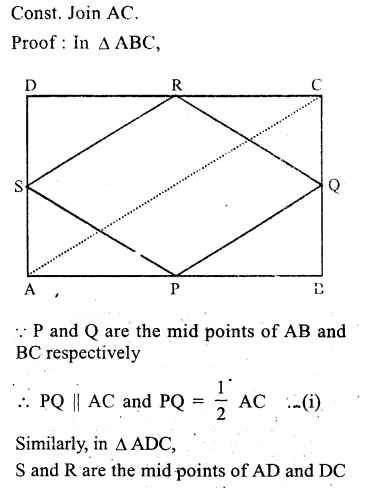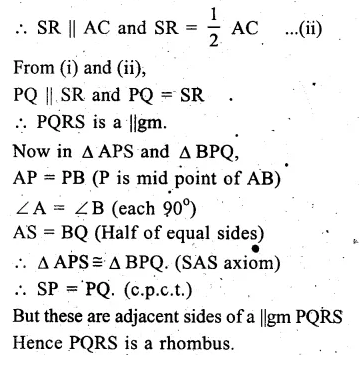Question 10.
Solution:
Given : In rhombus ABCD, P, Q, R and S are the mid points of sides AB, BC, CD and DA respectively PQ, QR, RS and SP are joined.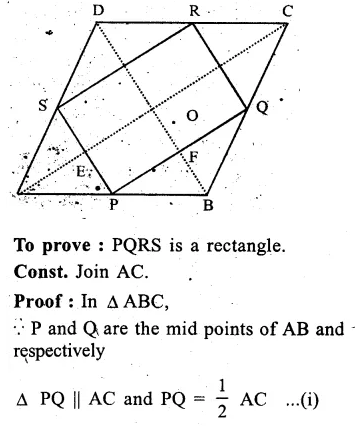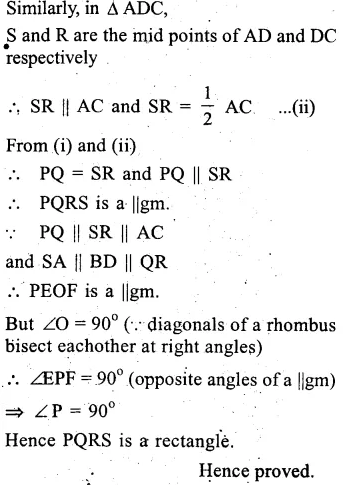Question 11.
Solution:
Given : In square ABCD, P,Q,R and S are the mid points of sides AB, BC, CD and DA respectively. PQ, QR, RS and SP are joined.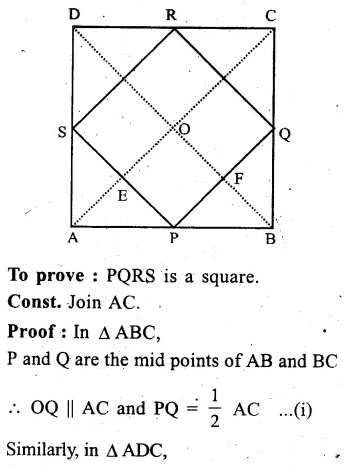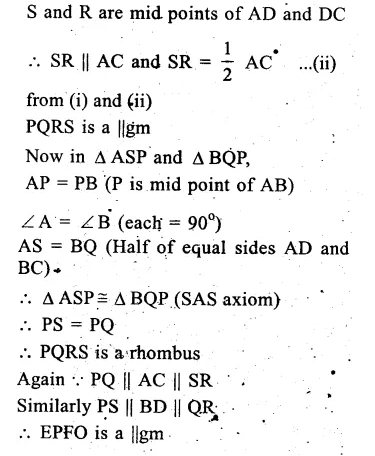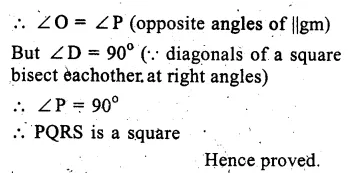Question 12.
Solution:
Given : In quadrilateral ABCD, P, Q, R and S are the midpoints of PQ, QR, RS and SP respectively PR and QS are joined.
To prove : PR and QS bisect each other
Const. Join PQ, QR, RS and SP and AC
Proof : In ∆ ABC,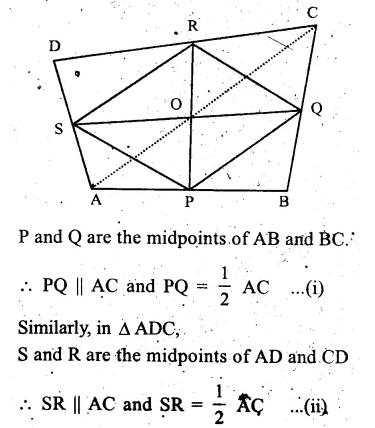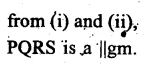But diagonals of a ||gm bisect each other PR and QS bisect each other.
∴ PR and QS bisect each other

Question 13.
Solution:
Given : ABCD is a quadrilateral. Whose diagonals AC and BD intersect each other at O at right angles.
P, Q, R and S are the mid points of sides AB, BC, CD and DA respectively. PQ, QR, QS and SP are joined.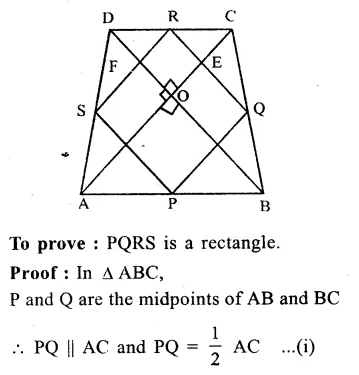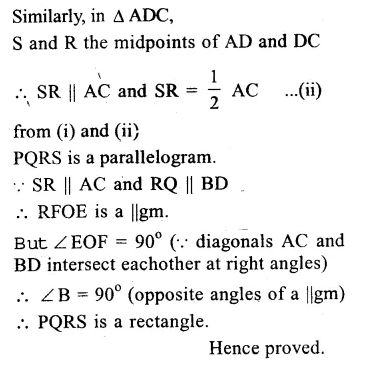Hope given RS Aggarwal Class 9 Solutions Chapter 9 Quadrilaterals and Parallelograms Ex 9C are helpful to complete your math homework.

If you have any doubts, please comment below. Learn Insta try to provide online math tutoring for you.